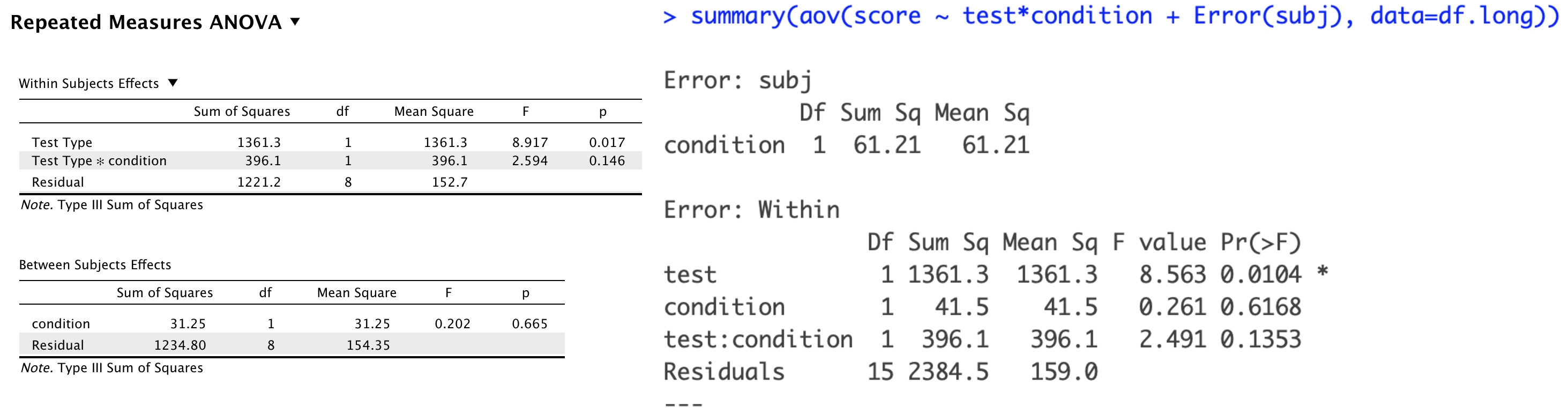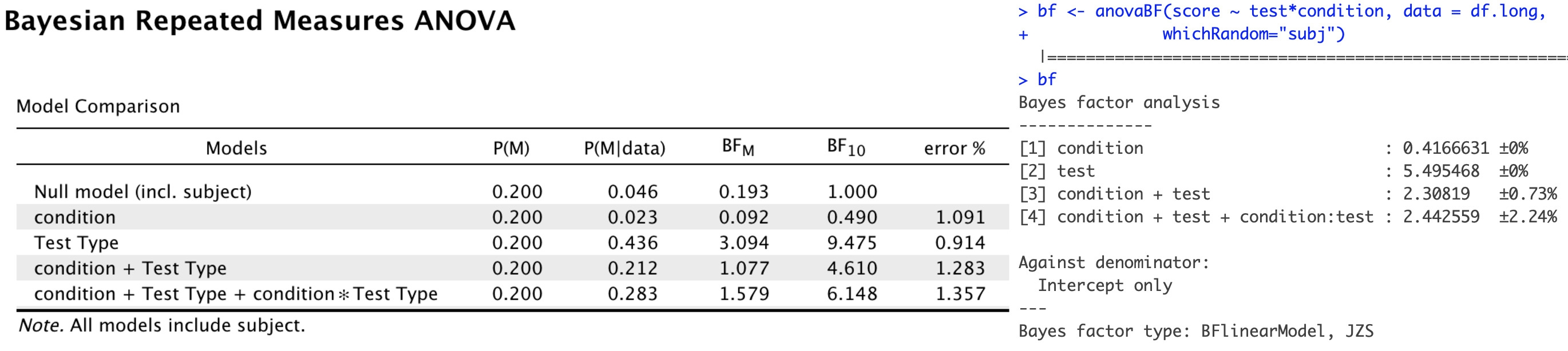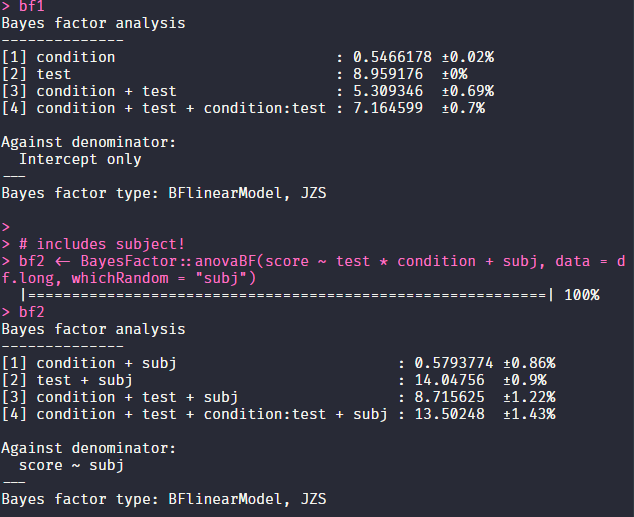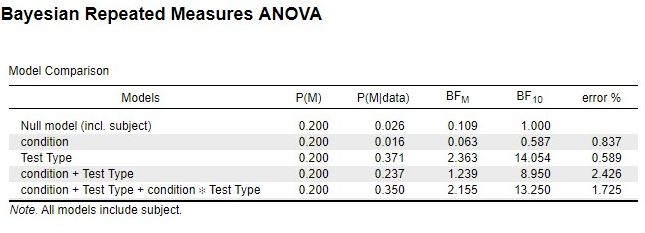#### Howdy, Stranger!

It looks like you're new here. If you want to get involved, click one of these buttons!

Supported by

# Discrepant results between JASP &amp; BayesFactor - Repeated Measures ANOVA

edited January 30

subj = 1:10,
Hey all

I am finding different results between the two when conducting a bayesian repeated measures anova, and I'm not sure if I'm mis-specifying it (in JASP or BayesFactor) or interpreting the output incorrectly.

Example experiment: Subjects are assigned conditions (treatment vs. control) and take two tests (science and math).

``````df <- data.frame(
subj = 1:10
condition = sample(x=c("treatment", "control"), size = 10, replace = T)
science.score = sample(x = 50:100,size =10, replace = T)
math.score = sample(x = 25:75,size =10, replace = T))

df.long <- melt(df,
variable.name = "test",
value.name = "score",
id.vars = c("subj", "condition"))
``````

When I perform a frequentist repeated measures anova in JASP and BayesFactor, the results converge:However, when I do a Bayesian Repeated Measures ANOVA, the results diverge (or, at least I think they do):I specified both ANOVAs the same way in JASP, so I'm not sure what I'm doing wrong!

Any help would be much appreciated

• The difference in the analysis is that JASP compares all models to the Null model that includes subject.

To replicate the results in R you would have to include "subj" into the model as well. In your example, it wasn't included which you can see from the "Against denominator: Intercept only"

A reproducible example:

``````set.seed(42)
df <- data.frame(
subj          = factor(1:10),
condition     = sample(x=c("treatment", "control"), size = 10, replace = T),
science.score = sample(x = 50:100,size =10, replace = T),
math.score    = sample(x = 25:75,size =10, replace = T)
)

df.long <- reshape2::melt(df,
variable.name = "test",
value.name    = "score",
id.vars       = c("subj", "condition")
)

summary(aov(score ~ test * condition + Error(subj), data = df.long))

# does not include subject!
bf1 <- BayesFactor::anovaBF(score ~ test * condition, data = df.long, whichRandom = "subj")
bf1

# includes subject!
bf2 <- BayesFactor::anovaBF(score ~ test * condition + subj, data = df.long, whichRandom = "subj")
bf2
``````

The results in R:The results in JASP:There is still a small numerical discrepancy, but that is nothing to worry about. I hope that solves the issue!

Thanked by 3EJ MAgoJ butlerbj
• awesome, thank you @vandenman for the help!